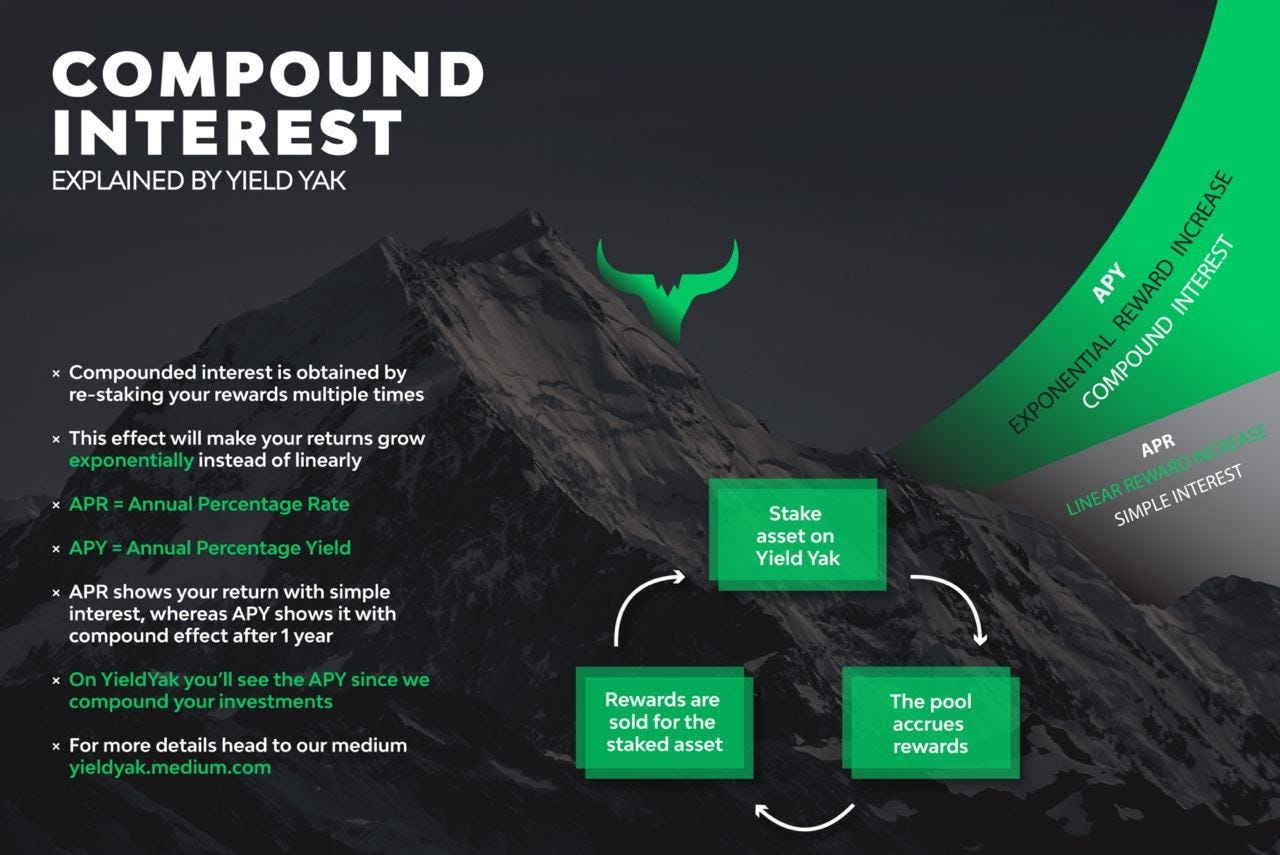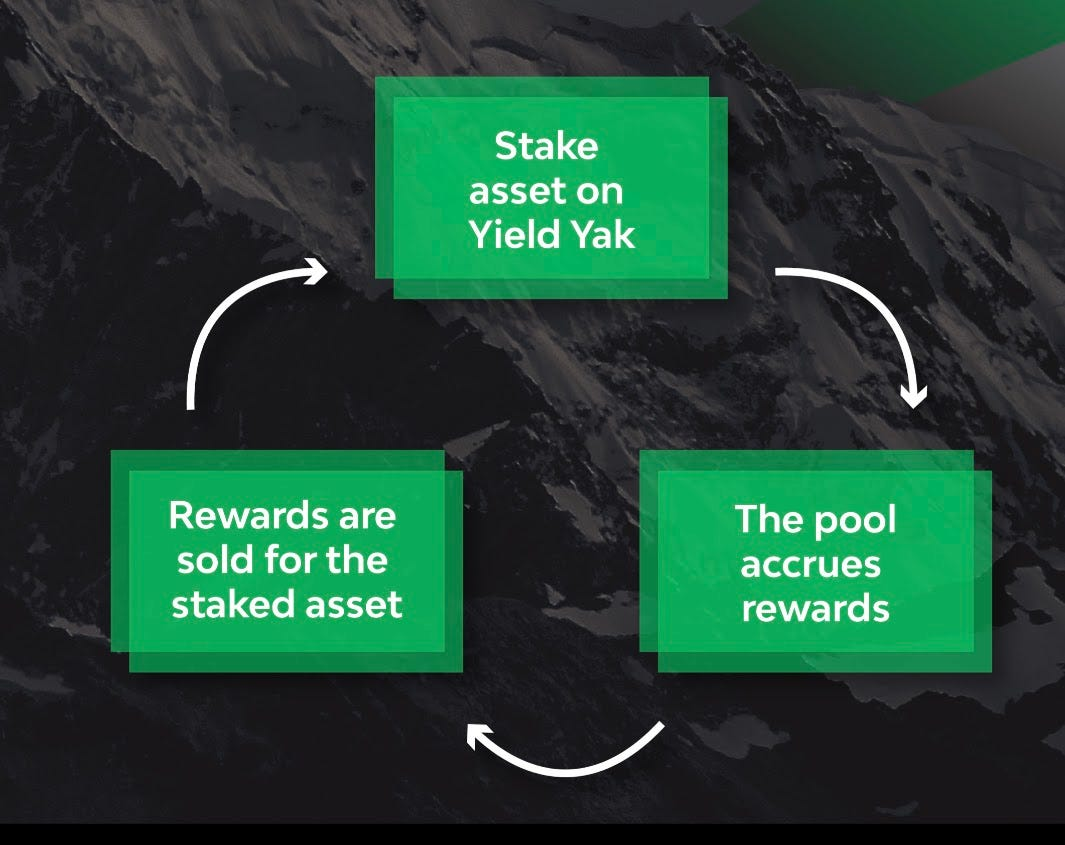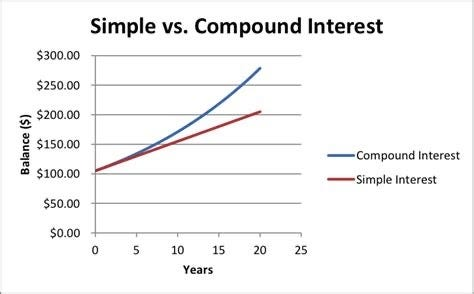💸

# Compound Interest

Yield Yak turns APR into APY, compounding your returns for more yieldThere is often a lot of confusion around the concept of APR, APY and compound interest. But worry not, our experienced compoundoors and Yak Guides are here to shed some light on these concepts for you.

## Compound interest: where the magic happens

What Yield Yak’s strategies do efficiently and effectively is compound your investments, but what does that mean exactly?
Each time you receive rewards from a farm, Yield Yak exchanges those rewards for the base asset and adds it to your position. This means that your next injection of rewards will come from a larger base amount.
Doing this repeatedly generates growth that accelerates over time: that is compound interest.The virtuous cycle of compound interest which makes investments grow EXPONENTIALLY
Compounding your rewards will make your returns increase exponentially instead of linearly, as you can see in this graph.Showing the difference between Simple Interest (APR) and Compound Interest (APY) over time
For shorter-term farmers, the effect of compound interest is relatively small compared to longer-term farmers. This is because the compounding effect creates a bigger gap over time. In both cases, Yield Yak offers a very convenient way to farm that efficiently converts farm incentives into yield.

## APR and APY: what’s the difference?

APR stands for Annual Percentage Rate. APR shows you the return on your investment without compounding.
APY stands for Annual Percentage Yield. APY shows you the return on your investment with compounding.
APR = plain annual return, APY = annual return with compound interest.
Generally, when you review a farm (like Pangolin or Trader Joe), you should consider the displayed figures in terms of APR because they do not compound your rewards.

### How to calculate APY from APR

For our more math-inclined friends, here’s how to calculate APY from APR yourself:
1. 1.
Divide APR by 100 and by 365 to know the daily return (assuming a daily reinvestment)
2. 2.
3. 3.
Raise the result to the power of 365 (assuming a daily reinvestment)
4. 4.
Subtract 1 from the result
5. 5.
Multiply by 100 and that’s your APY!
Note: You can also check periods other than daily, for example replace 365 with 12 for monthly. Or 730 for twice-a-day.
Let’s try an example: if you put \$100 in a farm with an APR of 50% then the APY would be:
1. 1.
50% APR/100 = 0.5 → 0.5/365 = 0.001369
2. 2.
0.001369+1 = 1.001369
3. 3.
1.001369³⁶⁵ = 1.6476
4. 4.
1.6476–1 = 0.6476
5. 5.
0.6476*100 = 64.76% APY
So if you didn’t compound you would receive \$50 in a year, whereas compounding you receive \$64.76.
And that’s it! The mystery behind compound interest and APY has been solved.